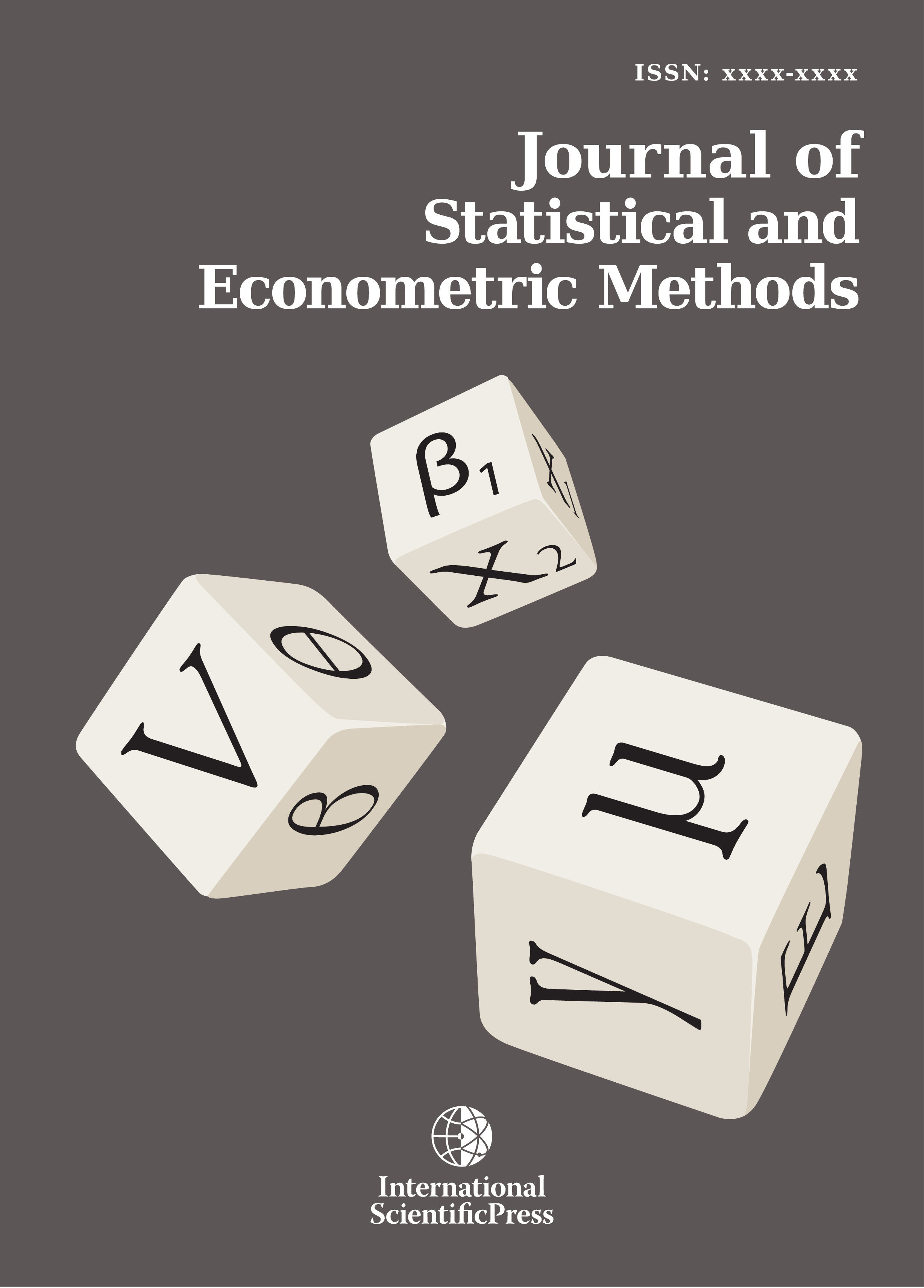# Journal of Statistical and Econometric Methods

#### Time-Varying Cross-Hedge Effectiveness: A Local Cointegration Approach

•[ Download ]
• Times downloaded: 1074
• Abstract

The dynamic nature of many asset price processes and the lack of perfect hedging assets can lead to unstable hedge ratios over time, ne- cessitating the re-estimation and rebalancing of cross-hedges. Cross- hedging occurs when a portfolio or asset is hedged with a statistically related yet not identical underlying derivative. Ordinary Least Squares regression is an oft applied method for estimating constant minimum- variance hedge ratios to curb price volatility or manage a market-neutral porfolio. However, constant estimates are often unsuitable under cross- hedging where the dependence structure between the two assets change over time. Rather than traditional correlation-based hedging, this paper focuses on cointegration-based cross-hedging with respect to the equilibrium between asset prices. We apply and test the out-of-sample efficacy of models that enable the cointegrating vector, or hedge ratio between two nonstationary price series, to vary over time. Models are estimated across daily data for selected equity, bond and commodity pairs. Rolling- window regression, exponentially-weighted moving average and Dynamic Linear Models (Gaussian Linear State-Space Models) are investigated. Results show that time-varying parameter models have superior out-of- sample hedging performance compared to constant parameter methods. This  finding is confirmed through extensive Monte Carlo simulation. In practice, this reduction in basis risk comes with incurred transaction costs from routine hedge rebalancing.ISSN: 2241-0376 (Online)
2241-0384 (Print)